CBSE Class 10 Sample Paper for 2020 Boards - Maths Standard

Class 10
Solutions of Sample Papers for Class 10 Boards

## A TV reporter was given a task to prepare a report on the rainfall of the city Dispur of India in a particular year. After collecting the data, he analyzed the data and prepared a report on the rainfall of the city. Using this report, he drew the following graph for a particular time period of 66 days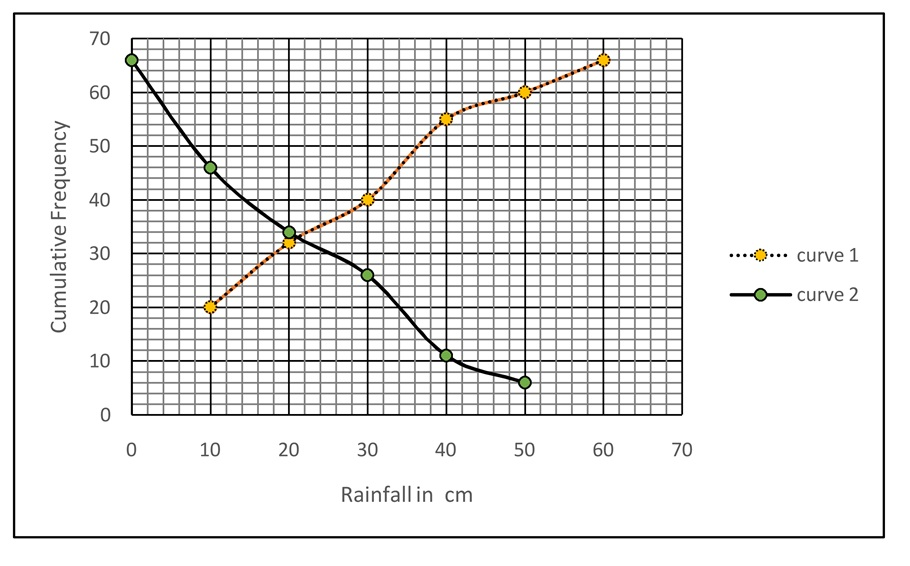## iii. Obtain the Mode of the data if mean rainfall is 23.4cm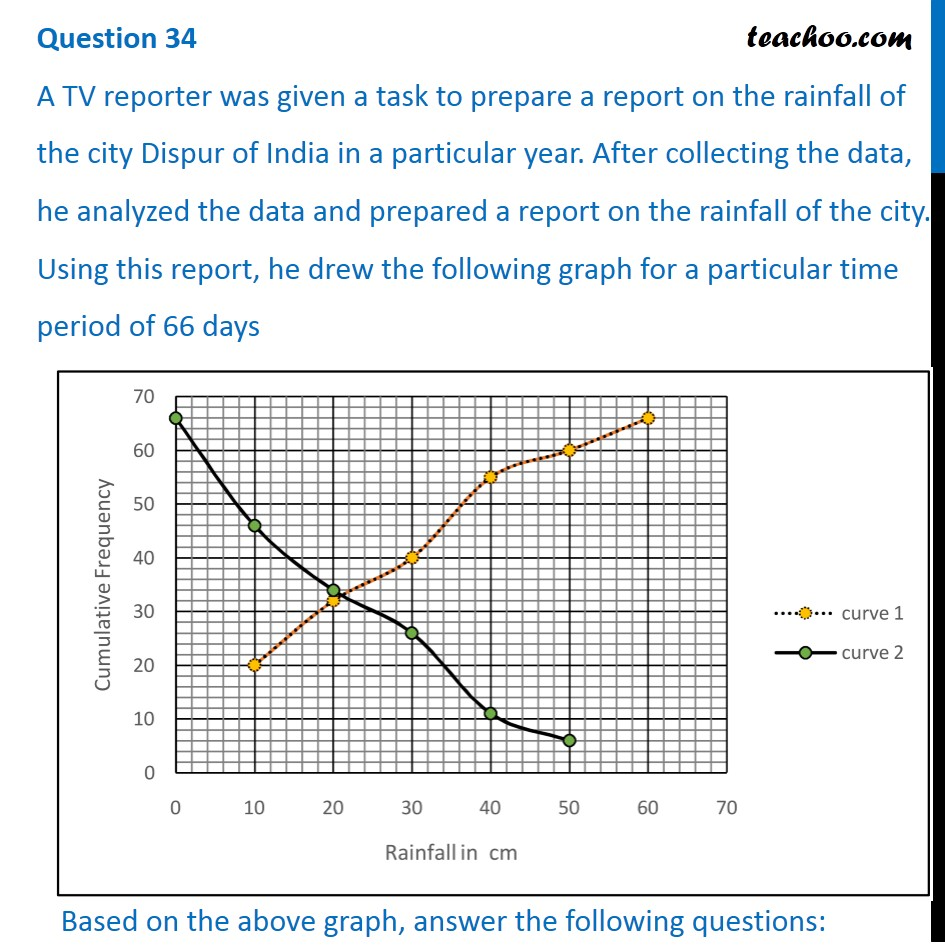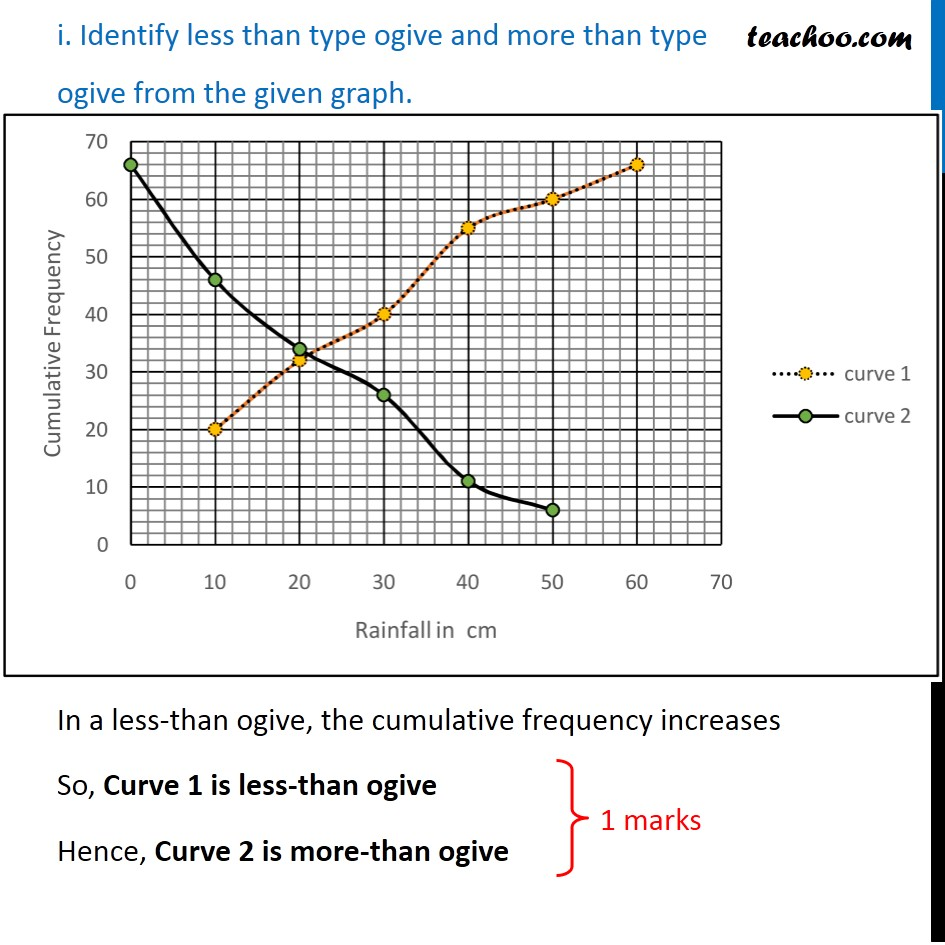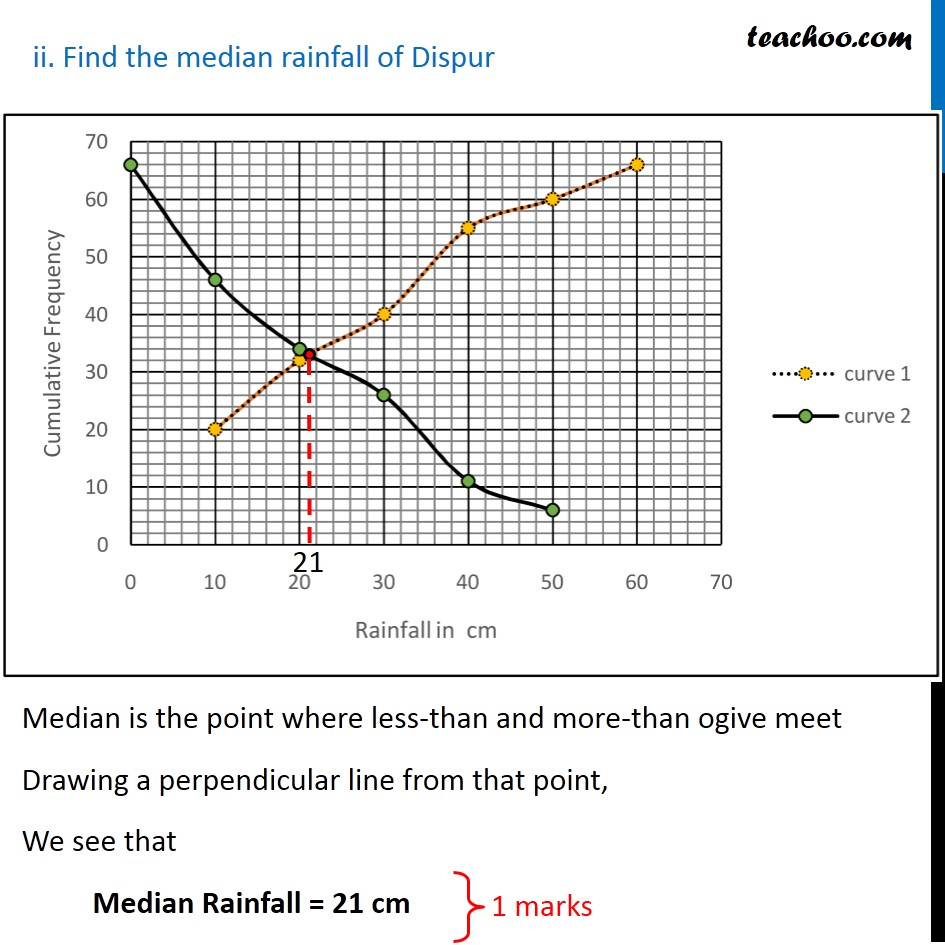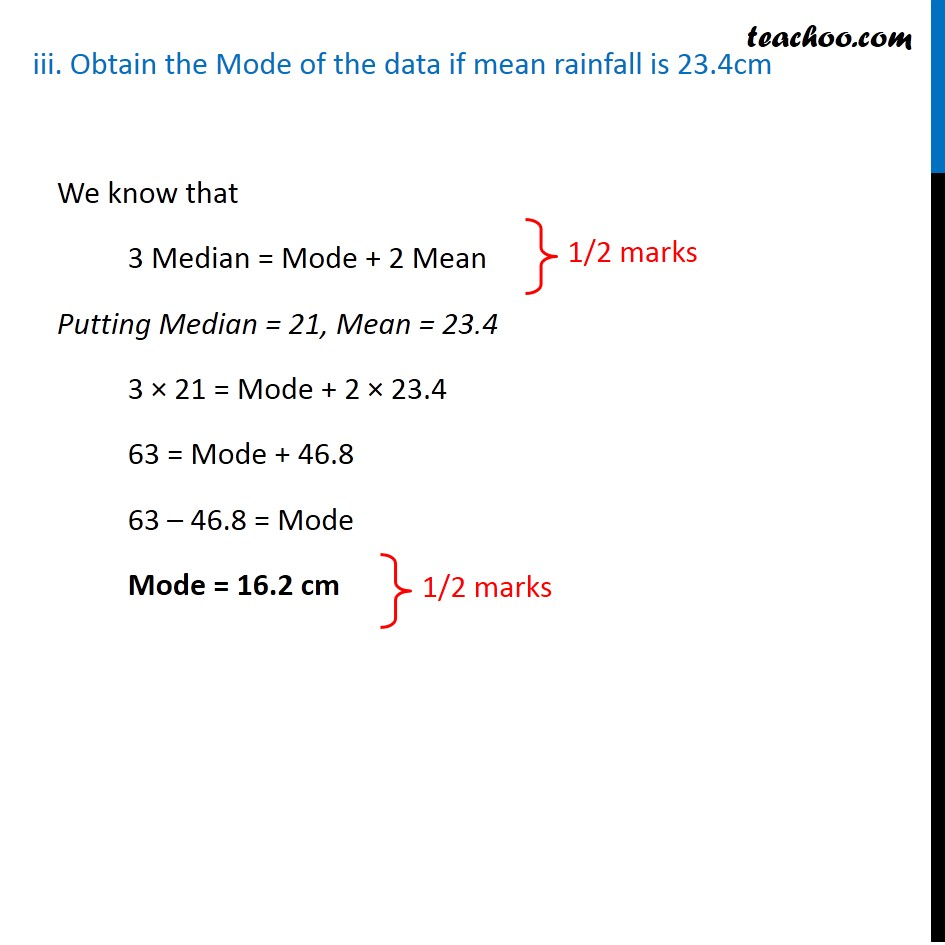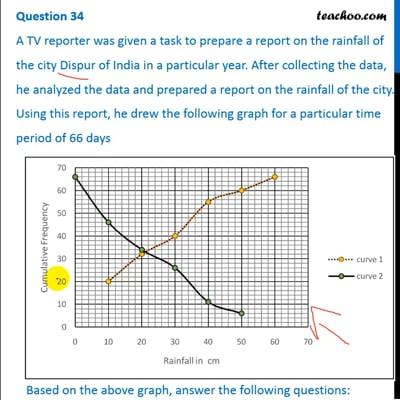This video is only available for Teachoo black users

Learn in your speed, with individual attention - Teachoo Maths 1-on-1 Class

### Transcript

Question 34 A TV reporter was given a task to prepare a report on the rainfall of the city Dispur of India in a particular year. After collecting the data, he analyzed the data and prepared a report on the rainfall of the city. Using this report, he drew the following graph for a particular time period of 66 days Based on the above graph, answer the following questions: i. Identify less than type ogive and more than type ogive from the given graph. In a less-than ogive, the cumulative frequency increases So, Curve 1 is less-than ogive Hence, Curve 2 is more-than ogive ii. Find the median rainfall of Dispur Median is the point where less-than and more-than ogive meet Drawing a perpendicular line from that point, We see that Median Rainfall = 21 cm iii. Obtain the Mode of the data if mean rainfall is 23.4cm We know that 3 Median = Mode + 2 Mean Putting Median = 21, Mean = 23.4 3 × 21 = Mode + 2 × 23.4 63 = Mode + 46.8 63 – 46.8 = Mode Mode = 16.2 cm